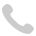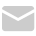国产非标准设备原价的构成和计算

1.费用构成：按成本计算估价法计算时，包括材料费、加工费、辅助材料费、专用工具费、废品损失费、外购配套件费、包装费、利润、税金和非标准设备设计费。

2.计算公式：{[(材料费+加工费+辅助材料费)×(1+专用工具费率)×(1+废品损失费率)+外购配套件费]×(1+包装费率)-外购配套件费}×(1+利润率)+销项税额+非标准设备设计费+外购配套件费。

3.注意事项：非标准设备原件有多种计算方法，如成本计算估价法、系列设备插入估价法、分部组合估价法、定额估价法等。成本计算估价法是一种比较常用的估算非标准设备原价的方法。

4.费用计算

(1)材料费=材料净重×(1+加工损耗系数) ×每吨材料综合价

(2)辅助材料费=设备总重量×辅助材料费指标

(3)加工费=设备总重量(吨)×设备每吨加工费

(4)专用工具费=(材料费+辅助材料费+加工费)×专用工具费率

(5)废品损失费=(材料费+辅助材料费+加工费)×(1+专用工具费率)×废品损失费率

(6)外购配套件费

(7)包装费=[(材料费+辅助材料费+加工费) ×(1+专用工具费率) ×(1+废品损失费率)+外购配套件费] ×包装费率

(8)利润={[(材料费+辅助材料费+加工费) ×(1+专用工具费率) ×(1+废品损失费率)+外购配套件费]×(1+包装费率)-外购配套件费｝×利润率

外购配套件费计取包装费，但不计取利润。

(9)税金，主要指增值税，通常是指设备制造厂销售设备时向购入设备方收取的销项税额。

当期销售税额=销售额×适用增值税率

销售额={[(材料费+辅助材料费+加工费) ×(1+专用工具费率) ×(1+废品损失费率)+外购配套件费]×(1+包装费率)-外购配套件费｝×(1+利润率)+外购配套件费

(10)非标准设备设计费

非标准设备设计费独立计算，与其他 9 项费用无关。

单台非标准设备原价={[(材料费+辅助材料费+加工费) ×(1+专用工具费率)×(1+废品损失费率)+外购配套件费] ×(1+包装费率)-外购配套件费}×(1+利润率)+外购配套件费+销项税额+非标准设备设计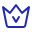VIP题库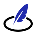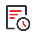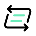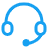帮助中心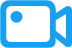关于我们扫码下载APP扫码关注公众号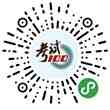扫码关注小程序